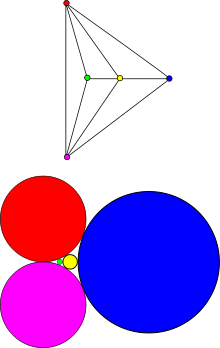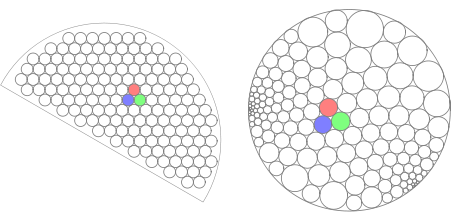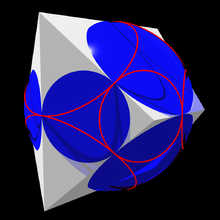# Circle packing theorem

﻿
Circle packing theoremExample of the circle packing theorem on K5, the complete graph on five vertices, minus one edge.

The circle packing theorem (also known as the Koebe–Andreev–Thurston theorem) describes the possible tangency relations between circles in the plane whose interiors are disjoint. A circle packing is a connected collection of circles (in general, on any Riemann surface) whose interiors are disjoint. The intersection graph (sometimes called the tangency graph or contact graph) of a circle packing is the graph having a vertex for each circle, and an edge for every pair of circles that are tangent. If the circle packing is on the plane, or, equivalently, on the sphere, then its intersection graph is called a coin graph. Coin graphs are always connected, simple, and planar. The circle packing theorem states that the converse also holds:

Circle packing theorem: For every connected simple planar graph G there is a circle packing in the plane whose intersection graph is (isomorphic to) G.

## A uniqueness statement

A graph G is triangulated planar if it is planar and every connected component of the complement of the embedding of G in the sphere has precisely three edges on its boundary, or in other words, if G is the 1-skeleton of a simplicial complex which is homeomorphic to the sphere. Any triangulated planar graph G is connected and simple, so the circle packing theorem guarantees the existence of a circle packing whose intersection graph is (isomorphic to) G. Such a G must also be finite, so its packing will have a finite number of circles. As the following theorem states more formally, every maximal planar graph can have at most one packing.

Koebe–Andreev–Thurston theorem: If G is a finite triangulated planar graph, then the circle packing whose tangency graph is (isomorphic to) G is unique, up to Möbius transformations and reflections in lines.

Thurston observes that this uniqueness is a consequence of the Mostow rigidity theorem. The plane in which the circles are packed may be viewed as the boundary of a halfspace model for hyperbolic space; with this view, each circle is the boundary of a plane within the hyperbolic space. One can define a set of disjoint planes from the circles of the packing, and a second set of disjoint planes defined by the circles surrounding each triangular gap between three of the circles in the packing. These two sets of planes meet at right angles, and form the generators of a reflection group whose fundamental domain can be viewed as a hyperbolic manifold. By Mostow rigidity, the hyperbolic structure of this domain is uniquely determined, up to isometry of the hyperbolic space; these isometries, when viewed in terms of their actions on the Euclidean plane on the boundary of the half-plane model, translate to Möbius transformations.

There is also a more elementary proof based on the maximum principle and on the observation that, in the triangle connecting the centers of three mutually tangent circles, the angle formed at the center of one of the circles is monotone decreasing in its radius and monotone increasing in the two other radii. Given two packings for the same graph G, one may apply reflections and Möbius transformations to make the outer circles in these two packings correspond to each other and have the same radii. Then, let v be an interior vertex of G for which the circles in the two packings have sizes that are as far apart as possible: that is, choose v to maximize the ratio r1/r2 of the radii of its circles in the two packings. For each triangular face of G containing v, it follows that the angle at the center of the circle for v in the first packing is less than or equal to the angle in the second packing, with equality possible only when the other two circles forming the triangle have the same ratio r1/r2 of radii in the two packings. But the sum of the angles of all of these triangles surrounding the center of the triangle must be 2π in both packings, so all neighboring vertices to v must have the same ratio as v itself. By applying the same argument to these other circles in turn, it follows that all circles in both packings have the same ratio. But the outer circles have been transformed to have ratio 1, so r1/r2 = 1 and the two packings have identical radii for all circles.

## Generalizations of the circle packing theorem

The circle packing theorem generalizes to graphs that are not planar. If G is a graph that can be embedded on a surface S, then there is a constant curvature Riemannian metric d on S and a circle packing on (Sd) whose contacts graph is isomorphic to G. If S is closed (compact and without boundary) and G is a triangulation of S, then (Sd) and the packing are unique up to conformal equivalence. If S is the sphere, then this equivalence is up to Möbius transformations; if it is a torus, then the equivalence is up to scaling by a constant and isometries, while if S has genus at least 2, then the equivalence is up to isometries.

Another generalization of the circle packing theorem involves replacing the condition of tangency with a specified intersection angle between circles corresponding to neighboring vertices. A particularly elegant version is as follows. Suppose that G is a finite 3-connected planar graph (that is, a polyhedral graph), then there is a pair of circle packings, one whose intersection graph is isomorphic to G, another whose intersection graph is isomorphic to the planar dual of G, and for every vertex in G and face adjacent to it, the circle in the first packing corresponding to the vertex intersects orthogonally with the circle in the second packing corresponding to the face.

Yet another variety of generalizations allow shapes that are not circles. Suppose that G = (VE) is a finite planar graph, and to each vertex v of G corresponds a shape$K_v\subset\mathbb R^2$, which is homeomorphic to the closed unit disk and whose boundary is smooth. Then there is a packing$P = (K'_v:v\in V)$ in the plane such that$K'_v\cap K'_u\ne \varnothing$ if and only if$[v,u]\in E$ and for each$v\in V$ the set K'v is obtained from Kv by translating and scaling. (Note that in the original circle packing theorem, there are three real parameters per vertex, two of which describe the center of the corresponding circle and one of which describe the radius, and there is one equation per edge. This also holds in this generalization.) One proof of this generalization can be obtained by applying Koebe's original proof and the theorem of Brandt and Harrington stating that any finitely connected domain is conformally equivalent to a planar domain whose boundary components have specified shapes, up to translations and scaling.

## Relations with conformal mapping theoryCircle packings can be used to approximate conformal mappings between specified domains. Each circle on the left corresponds to a circle on the right.

## Applications of the circle packing theorem

The circle packing theorem is a useful tool to study various problems in planar geometry, conformal mappings and planar graphs. An elegant proof of the planar separator theorem, originally due to Lipton and Tarjan, has been obtained in this way. Another application of the circle packing theorem is that unbiased limits of bounded-degree planar graphs are almost surely recurrent. Other applications include implications for the cover time. and estimates for the largest eigenvalue of bounded-genus graphs.

Circle packing has also become an essential tool in origami design, as each appendage on an origami figure requires a circle of paper. Robert J. Lang has used the mathematics of circle packing to develop computer programs that aid in the design of complex origami figures.

## Proofs of the theorem

There are many known proofs of the circle packing theorem. Paul Koebe's original proof is based on his conformal uniformization theorem saying that a finitely connected planar domain is conformally equivalent to a circle domain. There are several different topological proofs that are known. Thurston's proof is based on Brouwer's fixed point theorem. There is also a proof using a discrete variant of Perron's method of constructing solutions to the Dirichlet problem. Yves Colin de Verdière proved the existence of the circle packing as a minimizer of a convex function on a certain configuration space.

## Implications

Fáry's theorem, that every graph that can be drawn without crossings in the plane using curved edges can also be drawn without crossings using straight line segment edges, follows as a simple corollary of the circle packing theorem: by placing vertices at the centers of the circles and drawing straight edges between them, a straight-line planar embedding is obtained.A polyhedron and its midsphere. The circle packing theorem implies that every polyhedral graph can be represented as the graph of a polyhedron that has a midsphere.

A variation of the circle packing theorem asserts that any polyhedral graph and its dual graph can be represented by two circle packings, such that the two tangent circles representing a primal graph edge and the two tangent circles representing the dual of the same edge always have their tangencies at right angles to each other at the same point of the plane. A packing of this type can be used to construct a convex polyhedron that represents the given graph and that has a midsphere, a sphere tangent to all of the edges of the polyhedron. Conversely, if a polyhedron has a midsphere, then the circles formed by the intersections of the sphere with the polyhedron faces and the circles formed by the horizons on the sphere as viewed from each polyhedron vertex form a dual packing of this type.

## Algorithmic aspects

Collins & Stephenson (2003) describe a numerical relaxation algorithm for finding circle packings, based on ideas of William Thurston. The version of the circle packing problem that they solve takes as input a planar graph, in which all the internal faces are triangles and for which the external vertices have been labeled by positive numbers. It produces as output a circle packing whose tangencies represent the given graph, and for which the circles representing the external vertices have the radii specified in the input. As they suggest, the key to the problem is to first calculate the radii of the circles in the packing; once the radii are known, the geometric positions of the circles are not difficult to calculate. They begin with a set of tentative radii that do not correspond to a valid packing, and then repeatedly perform the following steps:

1. Choose an internal vertex v of the input graph.
2. Calculate the total angle θ that its k neighboring circles would cover around the circle for v, if the neighbors were placed tangent to each other and to the central circle using their tentative radii.
3. Determine a representative radius r for the neighboring circles, such that k circles of radius r would give the same covering angle θ as the neighbors of v give.
4. Set the new radius for v to be the value for which k circles of radius r would give a covering angle of exactly 2π.

Each of these steps may be performed with simple trigonometric calculations, and as Collins and Stephenson argue, the system of radii converges rapidly to a unique fixed point for which all covering angles are exactly 2π. Once the system has converged, the circles may be placed one at a time, at each step using the positions and radii of two neighboring circles to determine the center of each successive circle.

Mohar (1993) describes a similar iterative technique for finding simultaneous packings of a polyhedral graph and its dual, in which the dual circles are at right angles to the primal circles. He proves that the method takes time polynomial in the number of circles and in log 1/ε, where ε is a bound on the distance of the centers and radii of the computed packing from those in an optimal packing.

## History

The circle packing theorem was first proved by Paul Koebe. William Thurston rediscovered the circle packing theorem, and noted that it followed from the work of E. M. Andreev. Thurston also proposed a scheme for using the circle packing theorem to obtain a homeomorphism of a simply connected proper subset of the plane onto the interior of the unit disk. The Thurston Conjecture for Circle Packings is his conjecture that the homeomorphism will converge to the Riemann mapping as the radii of the circles tend to zero. The Thurston Conjecture was later proved by Burton Rodin and Dennis Sullivan. This led to a flurry of research on extensions of the circle packing theorem, relations to conformal mappings, and applications.

Wikimedia Foundation. 2010.

### Look at other dictionaries:

• Circle packing — This article describes the packing of circles on surfaces. For the related article on circle packing with a prescribed intersection graph, please see the circle packing theorem. The most efficient way to pack different sized circles together is… …   Wikipedia

• Packing problem — Part of a series on Puzzles …   Wikipedia

• List of circle topics — This list of circle topics includes things related to the geometric shape, either abstractly, as in idealizations studied by geometers, or concretely in physical space. It does not include metaphors like inner circle or circular reasoning in… …   Wikipedia

• Fáry's theorem — states that any simple planar graph can be drawn without crossings so that its edges are straight line segments. That is, the ability to draw graph edges as curves instead of as straight line segments does not allow a larger class of graphs to be …   Wikipedia

• Mostow rigidity theorem — In mathematics, Mostow s rigidity theorem, or strong rigidity theorem, or Mostow–Prasad rigidity theorem, essentially states that the geometry of a finite volume hyperbolic manifold of dimension greater than two is determined by the fundamental… …   Wikipedia

• List of mathematics articles (C) — NOTOC C C closed subgroup C minimal theory C normal subgroup C number C semiring C space C symmetry C* algebra C0 semigroup CA group Cabal (set theory) Cabibbo Kobayashi Maskawa matrix Cabinet projection Cable knot Cabri Geometry Cabtaxi number… …   Wikipedia

• Planar graph — Example graphs Planar Nonplanar Butterfly graph K5 The complete graph K4 …   Wikipedia

• William Thurston — Infobox Scientist name = William Thurston birth date = birth date and age|1946|10|30 birth place = death date = death place = residence = citizenship = nationality = American ethnicity = field = mathematics work institutions = Cornell University …   Wikipedia

• Geometric graph theory — In mathematics, a geometric graph is a graph in which the vertices or edges are associated with geometric objects or configurations. Geometric graph theory is a specialization of graph theory that studies geometric graphs. Notable geometric… …   Wikipedia

• Midsphere — A polyhedron and its midsphere. The red circles are the boundaries of spherical caps within which the surface of the sphere can be seen from each vertex. In geometry, the midsphere or intersphere of a polyhedron is a sphere which is tangent to… …   Wikipedia